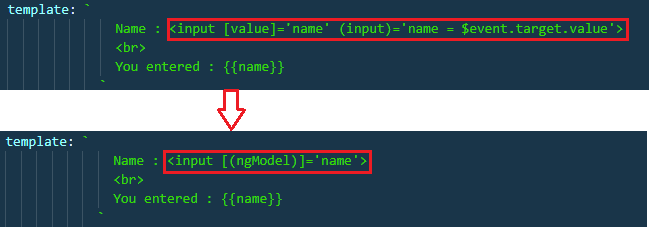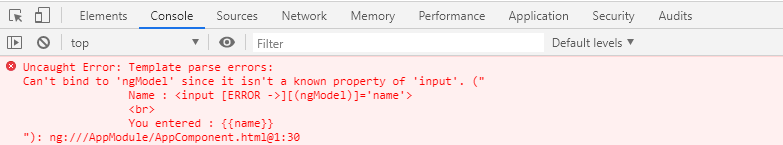• What is Angular Two Way Binding ?
• Example of Angular Two Way Data Binding.
What is Angular Two Way Binding ?

Two-way data binding in Angular will help users to exchange data from the component to view and from view to the component. It will help users to establish communication bi-directionally.

The two-way data binding is basically used in the input type filed or any form element where the user type or provide any value or change any control value on the one side and on the other side, the same automatically updated into the component variables and vice-versa is also true.Fig-1

The two-way data binding in Angular is a combination of Property Binding and Event Binding. The Syntax is given below:

<input [value]='name' (input)='name = \$event.target.value'>

Example of Angular Two Way Data Binding

In this article we discuss two way data binding in two ways.
•    Two-way-data-binding using the input event of the input control.
•    Two-way-data-binding using ngModel Directive.

➥ Two-way-data-binding using the input event of the input control

To binding to the input event of the input control the app.component.ts file is look like below;

Code ExplanationFig-2

<input [value]='name'> : Binds component class "name" property to the input element’s value property
You entered : {{name}} : Interpolation displays the value we have in "name" property on the web page.

At the moment when we change the value in the textbox, the changed value is not reflected in the browser. One way to achieve this is by binding to the input event of the input control as shown below.Fig-3
<input [value]='name' (input)='name = \$event.target.value'

When you run it again with the changes, the value is displayed as shown in [Fig-3]

Let's discuss what we have done in above;
• [value]='name' : This property binding flows data from the component class to element property
• (input)='name = \$event.target.value' : This event binding flows data in the opposite direction i.e from the element to component class property "name"
• \$event - Is exposed by angular event binding, and contains the event data. To retrieve the value from the input element use - \$event.target.value.
• name = \$event.target.value - This expression updates the value in the name property in the component class
• You entered : {{name}} - This interpolation expression will then display the value on the web page.
Here we see in two-way data binding Angular is a combination of both Property Binding and Event Binding. To save a few keystrokes and simplify two-way data binding angular has provided ngModel directive.

➥ Two-way-data-binding using the ngModel Directive

To simplify the two-way data binding, the angular framework has provided one directive called the ngModel directive. With the ngModel directive, you can change to existing code as shown below.Fig-4

With the above changes in place in the app.component.ts file, at this point, if you run the application, then you will get the following error.Fig-5
The error comes due to ngModel directive is available in the system module called FormsModule. If you want to use the ngModel directive, then in your root module that is AppModule, you will have to import the FormsModule first.

Here are the steps to import FormsModule into our AppModule

➜ Open app.module.ts file
➜ Include the following import statement in it
import { FormsModule } from '@angular/forms';
➜ Also, include FormsModule in the 'imports' array of @NgModule
imports: [BrowserModule, FormsModule]

With the above changes in place, the complete code of App.Module.ts is as follows.

The app.component.ts file as follows

With these changes, reload the web page and it will work as expected.

Syntax for using two-way data binding in Angular

<input [(ngModel)]='name'>
• The square brackets on the outside are for property binding
• The parentheses on the inside are for event binding
• To easily remember this syntax, compare it to a banana in a box [()]

Summary
In this tutorial we discussed Two way data binding in Angular. If have any question related to this topic then give your feedback.